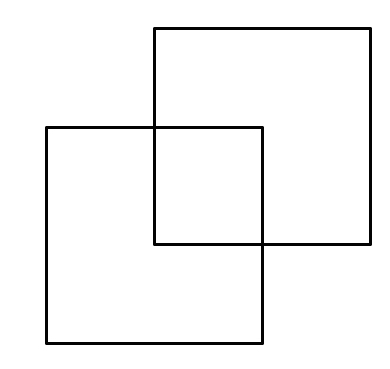# With Just 2 Squares

Probability Level 3What is the maximum number of regions that 2 unit squares can divide a plane into?

In the above images, we see that the 2 unit squares divide the plane into 4 regions: one overlapping region, two non-overlapping regions,and the region outside the two squares.

×